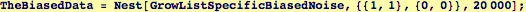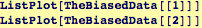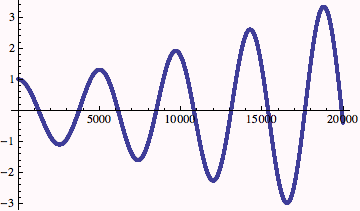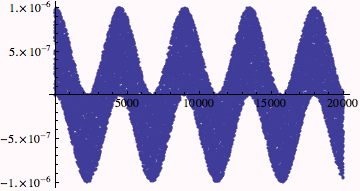Resonance phenomena simulated by finite differences and biased noise inputs

In Lecture-21.nb, a second-order differencing scheme that iteratively solved  y'' + βy' + γy=0  with the specification of two initial values.
Modify this to add a little random noise to y[i] at each step and observe how this behaves---this version will store the noise added at each iteration so that it can be visualized later....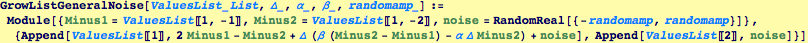Setting up a function that takes particular parameters and a "noise amplitude" of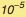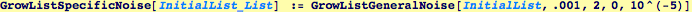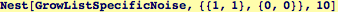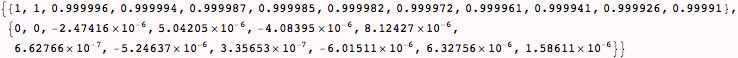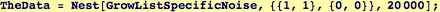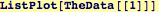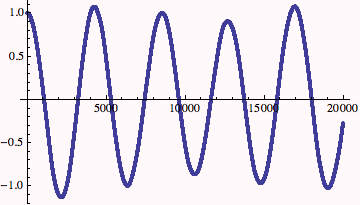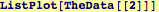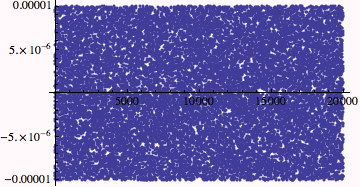Now suppose there is a periodic bias that tends to kick the displacement one direction more than the other: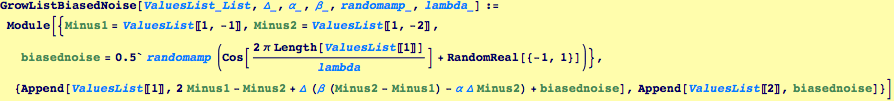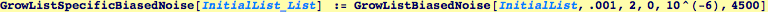Generate the data set---this takes quite a while